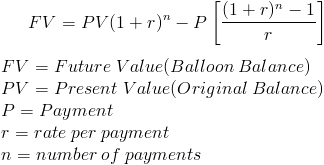# Balloon Balance of a LoanThe balloon loan balance formula is used to calculate the amount due at the end of a balloon loan.

A balloon loan, sometimes referred to as a balloon note, is a note that has a term that is shorter than its amortization. In other words, the loan payment will be amortized, or calculated, for a certain amount of years but the loan will be paid off before all payments calculated are made, thus leaving a balance due. An example would be a note that is calculated for 30 years, but the remaining balance after 10 years must be paid in one full sum. This example is commonly referred to as a 10/30 balloon.

The loan balloon balance formula can be used for any type of balloon loan and is commonly seen with mortgages and leases.

## Balloon Balance Formula and Remaining Balance Formula

The formula to calculate a balloon balance is the same formula used to calculate the remaining balance on a loan. The same formula is used because the amount due at the end of a balloon loan is effectively the same as calculating the balance of a conventional loan after the same period, all other things held constant.

## Example of Loan Balloon Balance Formula

An example of the loan balloon balance formula would be a \$100,000 5/15 balloon mortgage with a 6% annual rate compounded monthly. If the loan payment formula is used based on a 15 year amortization, the monthly payment would be \$843.86.

It is important to remember that private mortgage insurance, property taxes, and homeowner's insurance may be included when an individual makes a payment, but for this example, we are calculating the monthly payment for the loan itself. We are also assuming that the first payment is due one month from the start of the loan, or that the interest included in the closing costs was adjusted to accomodate this assumption.

For a 5/15 balloon, the loan will be amortized for 15 years, while we are solving for the amount due after the 5th year. The variables of the formula would be \$100,000 for present value (PV), \$843.86 for P (payment), .005 for the rate(the monthly rate for 6% per year), and 60 for the number of periods as there will be 60 months.

After putting these variables into the formula, the equation would beUsing this formula, the remaining balance would be \$76,008.88.

It must be taken into consideration that this remaining amount due would be after the 60th payment is made. For an individual that has a loan, they would need to pay the final payment as well as the balloon balance.

*Disclaimer:This site is compensated for sales made through affiliate links. There is no additional cost to you and it keeps the site running.

Resources

Financial Education ←

Increase your knowledge in Finance and other topics through courses at Udemy. Financial course topics include Personal Finance, Financial Analysis, Investing, Tech Analysis, and more.

Start Learning Today! Udemy courses up to 50% off (affiliate)

Banking/Investment ←

Invest your spare change (microinvesting), save for retirement, and get bonuses while you shop through Acorns

Start Now! Get a \$5 Sign Up Bonus for Acorns today! (Tier) (affiliate)

New to Finance?